Test: Semiconductor Physics

# Test: Semiconductor Physics

Test Description

## 10 Questions MCQ Test Analog Electronics | Test: Semiconductor Physics

Test: Semiconductor Physics for Electrical Engineering (EE) 2022 is part of Analog Electronics preparation. The Test: Semiconductor Physics questions and answers have been prepared according to the Electrical Engineering (EE) exam syllabus.The Test: Semiconductor Physics MCQs are made for Electrical Engineering (EE) 2022 Exam. Find important definitions, questions, notes, meanings, examples, exercises, MCQs and online tests for Test: Semiconductor Physics below.
Solutions of Test: Semiconductor Physics questions in English are available as part of our Analog Electronics for Electrical Engineering (EE) & Test: Semiconductor Physics solutions in Hindi for Analog Electronics course. Download more important topics, notes, lectures and mock test series for Electrical Engineering (EE) Exam by signing up for free. Attempt Test: Semiconductor Physics | 10 questions in 10 minutes | Mock test for Electrical Engineering (EE) preparation | Free important questions MCQ to study Analog Electronics for Electrical Engineering (EE) Exam | Download free PDF with solutions
 1 Crore+ students have signed up on EduRev. Have you?
Test: Semiconductor Physics - Question 1

### In the problems assume the parameter given in following table. Use the temperature T= 300 K unless otherwise stated.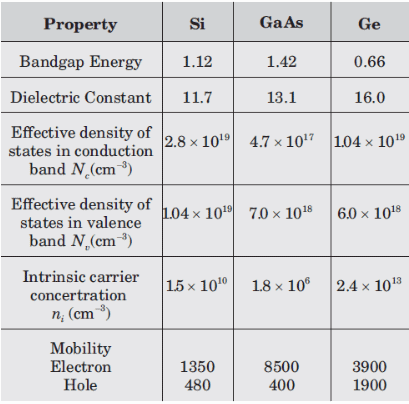In germanium semiconductor material at T 400 K the intrinsic concentration is (x 10^14 per cc)

Detailed Solution for Test: Semiconductor Physics - Question 1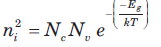Test: Semiconductor Physics - Question 2

### The intrinsic carrier concentration in silicon is to be no greater than ni = 1 x 10^12 cc. The maximum temperature allowed for the silicon is ( Eg = 1.12 eV)

Test: Semiconductor Physics - Question 3

### Two semiconductor material have exactly the same properties except that material A has a bandgap of 1.0eV and material B has a bandgap energy of 1.2 eV. The ratio of intrinsic concentration of material A to that ofmaterial B is

Detailed Solution for Test: Semiconductor Physics - Question 3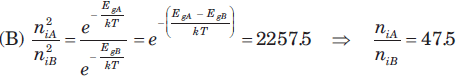Test: Semiconductor Physics - Question 4

In silicon at T = 300 K the thermal-equilibrium concentration of electron is n0 = 5 x 10^4 cc. The hole concentration is

Detailed Solution for Test: Semiconductor Physics - Question 4

ni x ni = no x po.

Test: Semiconductor Physics - Question 5

In silicon at T = 300 K if the Fermi energy is 0.22 eV above the valence band energy, the value of p0 is​

Detailed Solution for Test: Semiconductor Physics - Question 5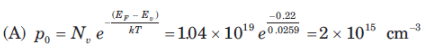Test: Semiconductor Physics - Question 6

The thermal-equilibrium concentration of hole p0 in silicon at T = 300 K is 1015 cm3. The value of n0 is

Detailed Solution for Test: Semiconductor Physics - Question 6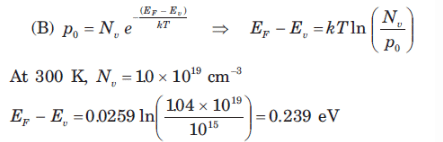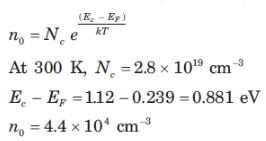Test: Semiconductor Physics - Question 7

In germanium semiconductor at T 300 K, the acceptor concentrations is Na 1013 cm3 and donor concentration is Nd 0. The thermal equilibrium concentration p0 is

Detailed Solution for Test: Semiconductor Physics - Question 7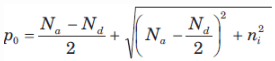Test: Semiconductor Physics - Question 8

A silicon sample doped n type at 10^18 cm3 have a resistance of 10 ohm. The sample has an area of 10^(-6) cm2 and a length of 10 µm . The doping efficiency of the sample is (µn = 800 cm2/V-s )

Detailed Solution for Test: Semiconductor Physics - Question 8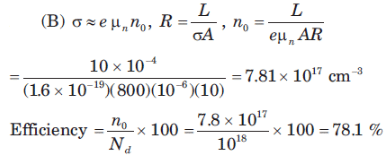Test: Semiconductor Physics - Question 9

Six volts is applied across a 2 cm long semiconductor bar. The average drift velocity is 104 cms. The electron mobility is

Detailed Solution for Test: Semiconductor Physics - Question 9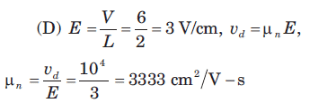Test: Semiconductor Physics - Question 10

A particular intrinsic semiconductor has a resistivity of 50 (ohm-cm) at T = 300 K and 5 (ohm-cm) at T = 330 K. If change in mobility with temperature is neglected, the bandgap energy of the semiconductor is

Detailed Solution for Test: Semiconductor Physics - Question 10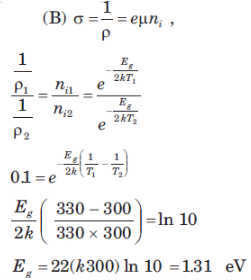## Analog Electronics

11 videos|57 docs|97 tests
 Use Code STAYHOME200 and get INR 200 additional OFF Use Coupon Code
Information about Test: Semiconductor Physics Page
In this test you can find the Exam questions for Test: Semiconductor Physics solved & explained in the simplest way possible. Besides giving Questions and answers for Test: Semiconductor Physics, EduRev gives you an ample number of Online tests for practice

## Analog Electronics

11 videos|57 docs|97 tests